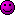# Thread: Determine if Now.TimeOfDay Is Between Two Times

1. ## Determine if Now.TimeOfDay Is Between Two Times

Hey Guys,

I want to make a function that determines if Now.TimeOfDay is between two times?

Basically I want to make a function to determine if the current time is between 6AM and 6PM, and return True if it is and False if its not.

I've been having a hard time doing the comparison. Any help would be appreciated.

Thx.Reply With Quote

2. ## Re: Determine if Now.TimeOfDay Is Between Two Times

Code:
```  Public Function IsDayTime() As Boolean
Return DateTime.Now.TimeOfDay >= New TimeSpan(6, 0, 0) AndAlso DateTime.Now.TimeOfDay <= New TimeSpan(18, 0, 0)
End Function```Reply With Quote

3. ## Re: Determine if Now.TimeOfDay Is Between Two TimesOriginally Posted by Joacim Andersson
Code:
```  Public Function IsDayTime() As Boolean
Return DateTime.Now.TimeOfDay >= New TimeSpan(6, 0, 0) AndAlso DateTime.Now.TimeOfDay <= New TimeSpan(18, 0, 0)
End Function```
How would you add two parameters to the function and pass the begin/end time that you wanted to check, and feed that into your function with the TimeSpan thing?Reply With Quote

4. ## Re: Determine if Now.TimeOfDay Is Between Two Times

Code:
```  Public Function IsDayTime(ByVal startTime As DateTime, ByVal endTime As DateTime) As Boolean
Return DateTime.Now.TimeOfDay >= startTime.TimeOfDay AndAlso DateTime.Now.TimeOfDay <= endTime.TimeOfDay
End Function```Reply With Quote

5. ## Re: Determine if Now.TimeOfDay Is Between Two Times

Ah......I had something like that going but I was trying to cram it into the TimeSpan thing, and that is where I was screwing myself up.

Thanks!Reply With Quote

6. ## Re: Determine if Now.TimeOfDay Is Between Two Times

Well TimeOfDay returns a TimeSpan. You could of course do it like this as well:
Code:
```  Public Function IsDayTime(ByVal startTime As DateTime, ByVal endTime As DateTime) As Boolean
Return DateTime.Now.TimeOfDay >= New TimeSpan(startDate.Ticks) AndAlso DateTime.Now.TimeOfDay <= New TimeSpan(endTime.Ticks)
End Function```
or if you want to be complicated:
Code:
```  Public Function IsDayTime(ByVal startTime As DateTime, ByVal endTime As DateTime) As Boolean
Return DateTime.Now.TimeOfDay >= New TimeSpan(startTime.Hours, startTime.Minutes, startTime.Seconds) AndAlso ...
End Function```Reply With Quote

7. ## Re: Determine if Now.TimeOfDay Is Between Two TimesNah, thats OK. The first way works just fine.Reply With Quote

####Posting Permissions

• You may not post new threads
• You may not post replies
• You may not post attachments
• You may not edit your posts
•

Featured

Click Here to Expand Forum to Full Width